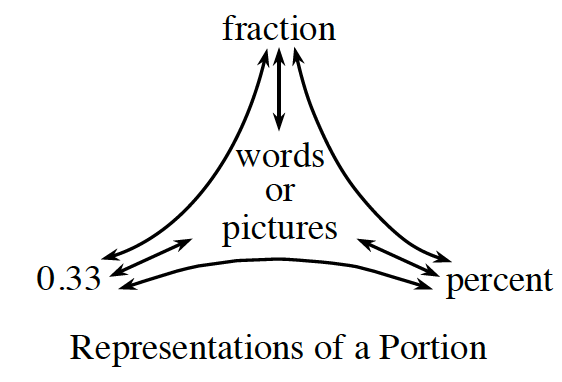### Home > CC1MN > Chapter 9 > Lesson 9.3.2 > Problem9-98

9-98.

Complete the web at right to represent $0.33$ as a percent, as a fraction, and in words.For help converting a decimal to a percent and a fraction, refer to the Math Notes box in Lesson 3.1.5.

$0.33$ is equal to $33\%$. Were you able to find a fraction to represent this? Don't forget to represent $0.33$ in words!